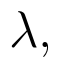Select Page

# CBSE Maths 12 Science Three Dimensional Geometry MCQ Answers in English

CBSE Maths 12 Science Three Dimensional Geometry MCQ Answers in English to enable students to get Answers in a narrative video format for the specific question.

Expert Teacher provides CBSE Maths 12 Science Three Dimensional Geometry MCQ Answers through Video Answers in English language. This video solution will be useful for students to understand how to write an answer in exam in order to score more marks. This teacher uses a narrative style for a question from Three Dimensional Geometry not only to explain the proper method of answering question, but deriving right answer too.

Please find the question below and view the Answer in a narrative video format.

Question:

Answer Video in English:

## Similar Questions from CBSE, 12th Science, Maths, Three Dimensional Geometry

Question 1 : Find the distance of the point (3, 4, 5) from the plane x + y + z = 2 measured parallel to the line 2x = y = z. (View Answer Video)

Question 2 : Find the intercepts cut off by the plane 2x + y - z = 5. (View Answer Video)

Question 3 : If the cartesian equations of a line arewrite the vector equation for the line. (View Answer Video)

Question 4 : Write the sum of intercepts cut off by the planeon the three axes.  (View Answer Video)

Question 5 : If the linesandare perpendicular to each other, then find the value of P. (View Answer Video)

### Matrices

Question 1 : Compute:. (View Answer Video)

Question 2 : Compute:. (View Answer Video)

Question 3 : Find the value of y from the equation:(View Answer Video)

Question 4 : Find the value of z,  from the equation:. (View Answer Video)

Question 5 :  Find the value of z, if(View Answer Video)

### Vector Algebra

Question 1 : Find the position vector of a point which divides the join of points with position vectorsandexternally in the ration 2:1.  (View Answer Video)

Question 2 : Find the unit vector in the direction of vector PQ, where P and Q are the points (1, 2, 3) and (4, 5, 6) respectively. (View Answer Video)

Question 3 : Findif the vectorsandare coplanar.   (View Answer Video)

Question 4 :  Find the scalar quantities from the following:
(i) 10 kg          (ii) 2 m north-west          (iii)(iv) 40 W         (v)(vi)(View Answer Video)

Question 5 : Find the vector quantities from the following:
(i) 10 kg          (ii) 2 m north-west          (iii)(iv) 40 W         (v)(vi)(View Answer Video)

### Integrals

Question 1 : Find the integral of the function. (View Answer Video)

Question 2 : Find the integral of the function. (View Answer Video)

Question 3 : Find. (View Answer Video)

Question 4 : Write the value of :(View Answer Video)

Question 5 : Show that :(View Answer Video)